# Primary 6 PSLE Practice #3

Time elapsed:
Q1. Alex bought a plant that was 16 cm tall. He measured the height of the plant and recorded its increase in height by the end of each week. The bar graph below shows his records. What was the height of the plant at the end of Week 3?     [1 mark]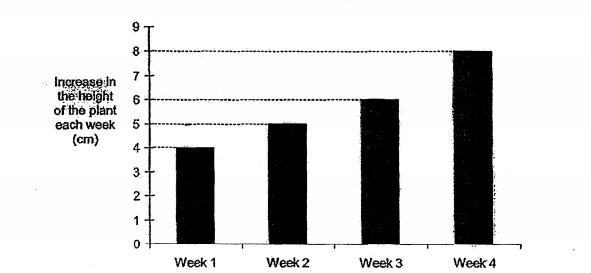Q2. Which one of the following can be the net of the solid shown below?     [2 marks]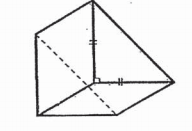Q3. A group of friends shared some chocolates among themselves. They tried taking 10 chocolates each, but found that the last person had only 2 chocolates. When each person took 8 chocolates, there were 20 left over. How many friends shared the chocolates?     [2 marks]
Q4. Express 2080 cm in m.     [1 mark]
Q5. What is the lowest common multiple of 6 and 8?     [1 mark]
Q6. In the diagram below, not drawn to scale, ACD is an equilateral triangle and BCD is a straight line. Find ∠y.     [1 mark]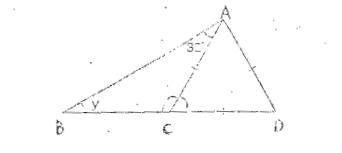Q7. The figure below shows a line DE and 4 points, L, M, N and P. Which point will form an angle greater than 45° but smaller than 90° at point E?     [1 mark]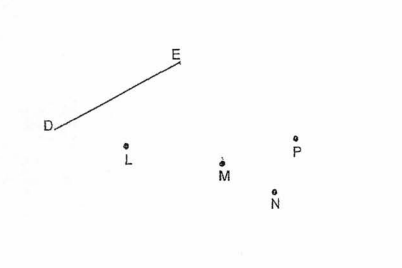Q8. Express 56 ÷ 2 in its simplest form.     [1 mark]
Q9. Xavier paid \$900 for a laptop at a discount of 10% at an electronics shop. He signed up as a member of the shop and enjoyed an additional 10% discount on top of the discounted price. What was the total discount Xavier received for buying the laptop?     [2 marks]
Q10. A motorist travelled at 60 km/h for the first 16 h. Then he travelled at 90 km/h for another 13 h. What was the total distance he travelled?     [2 marks]
Q11. The empty cuboid below measures 8 cm by 9 cm by 15 cm. Find the area of the shaded face.     [1 mark]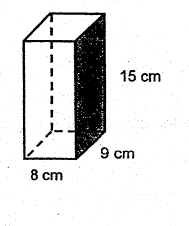Q12. Victor purchased 50 identical toy cars at a discount of 30%. Kingston purchased the same number of such toy cars but was given only a discount of 20%. If Kingston paid \$960 for the toy cars, how much did Victor pay for his toy cars?     [2 marks]
Q13. In the diagram, J, K and L are three points on the ground. Point J is north of point K and ∠JKL is 315°. In what direction is point L from point K?     [1 mark]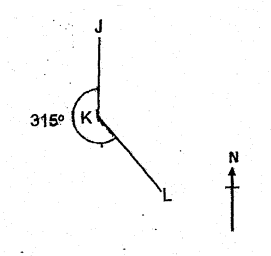Q14. Which one of the following is smaller than 38?     [1 mark]
Q15. A string was used to from Square X. The string was then used to form Figure Y with three straight sides and one curved side. Square X has the same perimeter as Figure Y with AB = BC = CD = 10 cm. Find the length of the curved side AD.     [1 mark]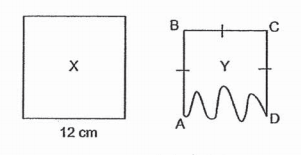Q16. Which of the following is the most likely height of the desk in a classroom?     [1 mark]
Q17. A block of wood measures 42 cm by 24 cm by 19 cm. What is the maximum number of cuboids with dimensions of 6 cm by 4 cm by 2 cm that can be cut from the piece of wood?     [1 mark]
Q18. Which of the following numbers has no remainder when it is divided by 4?     [1 mark]
Q19. What is the value of 5k - 3k/2 when k = 6?     [1 mark]
Q20. Mark, Paul and Shawn have some marbles. The ratio of the number of marbles Mark has to the number or marbles Paul has is 2 : 7. The total number of marbles Mark and Paul have is three times as many as the number of marbles Shawn has. Mark and Shawn have 300 marbles. How many marbles does Paul have?     [2 marks]